## Example Questions

### Example Question #1 : How To Add Decimals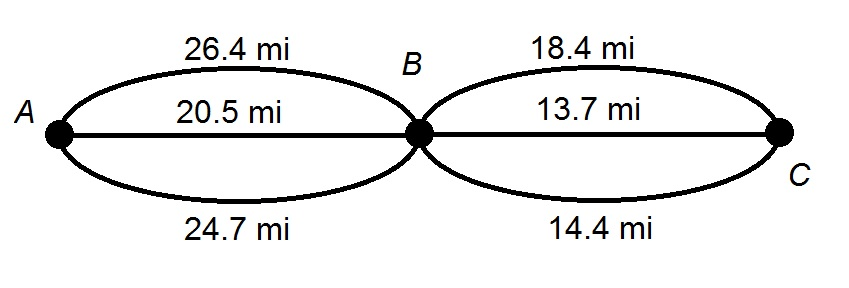A family is taking a trip from Town A to Town B, then to Town C. Above is a diagram of the routes available to them.

Give the range for the driving distancefor the trip.

None of the other choices gives the correct response.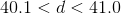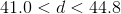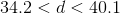Explanation:

Each route includes one path from Point A to Point B and one path from Point B o Point C.

The shortest possible drive is the sum of the shortest paths for each leg of the trip: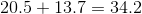The longest possible drive is the sum of the longest paths for each leg of the trip: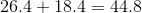The correct response is that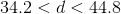.

### Example Question #1 : How To Add DecimalsAbove is a simplified map of the routes from Town A to Town B, and the routes from Town B to Town C.

A family wants to travel from Town A to Town C by way of Town B, then back to Town A by way of Town B. Since all routes are scenic, the family does not want to take any route twice.

Give the range for the distancein miles that the family will travel.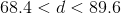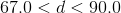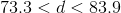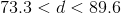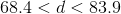Explanation:

The family's trip will be designed so that the family will take two different routes of the three that connect Town A and Town B, and two different routes of the three that connect Town B and Town C.

The minimum distance that the family will travel is therefore the sum of the lengths of the two shortest routes from Town A to Town B, and those of the two shortest routes from Town B to Town C: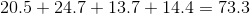miles

The maximum distance that the family will travel is, similarly, the sum of the lengths of the two longest routes from Town A to Town B, and those of the two longest routes from Town B to Town C: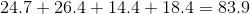miles

The correct choice is therefore.

### Example Question #73 : DecimalsA family is taking a trip from Town A to Town B, then to Town C. Above is a diagram of the routes available to them. How many routes will only require them to drive 40 miles or fewer:

Five

Four

Six

Three

Two

Five

Explanation:

There are three routes from Point A to Point B, and three from Point B to Point C, for a total of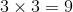routes total. The total distance traveled is the distance of one of the first three routes added to that of one of the last three; we can take all nine possibilities and add the distances: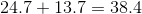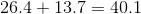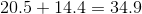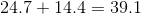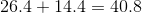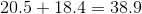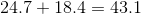Five of these routes require driving a distance 40 miles or fewer.

### Example Question #21 : Decimals

Round the answer to the nearest tenth.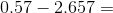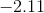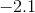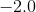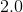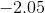Explanation:

0.57 - 2.657 = -2.087

Don't forget the negative sign!

This number then rounds to -2.1.

### Example Question #1 : How To Multiply Decimals

45.728 x 3.2 = ?

1463.296

146329.6

14.63296

146.3296

14632.96

146.3296

Explanation:

Multiply the numbers out in long format, then move the decimal point over the total number of decimal points in the two numbers (3 in the first, 1 in the second, so 4 total):

45.728 * 3.2 = 91456

91456 + 1371840 = 1,463,296 → 146.3296

Or just count the decimal points in the answer and make sure it's 4.

### Example Question #492 : Arithmetic

At the farmer's market, oranges are $0.30 each, apples are$0.25 each, bananas are $0.40 each, and tomatoes are$0.60 each. If Scott buys 3 oranges, 7 apples, 4 bananas, and 8 tomatoes, how much does he spend?

$11.40$6.80

$10.40$7.75

$9.05 Correct answer:$9.05

Explanation:

To find out how much Scott spends, we need to multiply the quantity of each fruit by its price and add them all up.

3 * $0.30 + 7 *$0.25 + 4 * $0.40 + 8 *$0.60 = \$9.05

### Example Question #1 : How To Multiply Decimals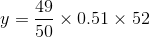Ifis 25% of, what is the closest integer to the value of?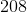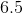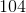Explanation:

We can approximate the value of y pretty quickly:

y ≈ 1(0.50)(52) = 26

Since y = 0.25x = (1/4)x we know that x  = 4y ≈ 4(26) = 104

### Example Question #11 : Decimals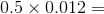6

0.0006

0.06

0.006

0.6

0.006

Explanation:

It's much easier to work with whole numbers, so we'll ignore the decimals for the first step: 5 x 12 = 60

Now look at the decimals. To add them back in, think of 60 as 60.0. Now move the decimal point four places to the left: 0.5 x 0.012 = .0060 = .006

### Example Question #11 : Decimal Operations

You bought a dozen eggs marked at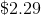and received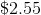change from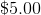. What is the percent of sales tax?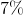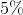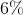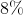Explanation:

Set the equation up as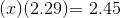Solve for, which equals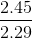or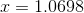Therefore the percent sales tax is: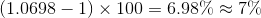### Example Question #71 : Decimals

simplify: 0.2 ÷ 0.04

5
0.005
0.02
0.05
2